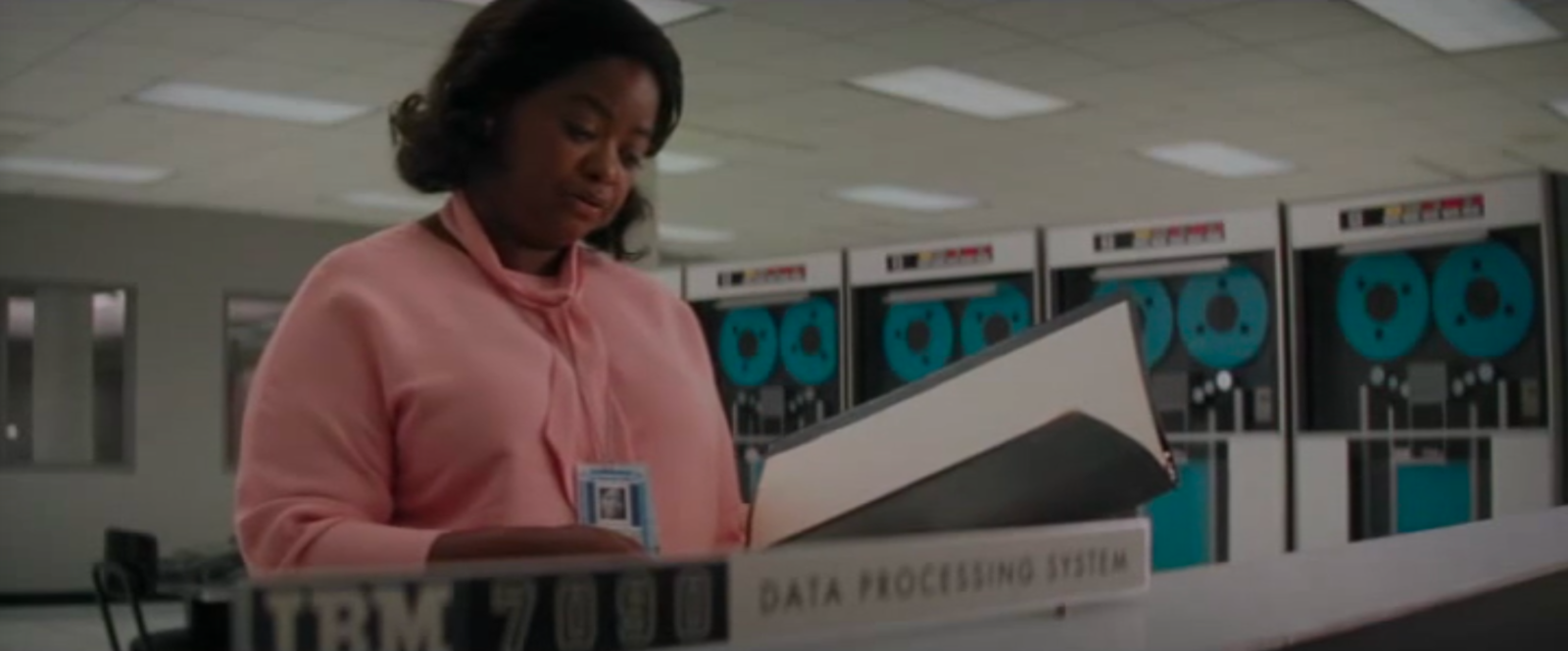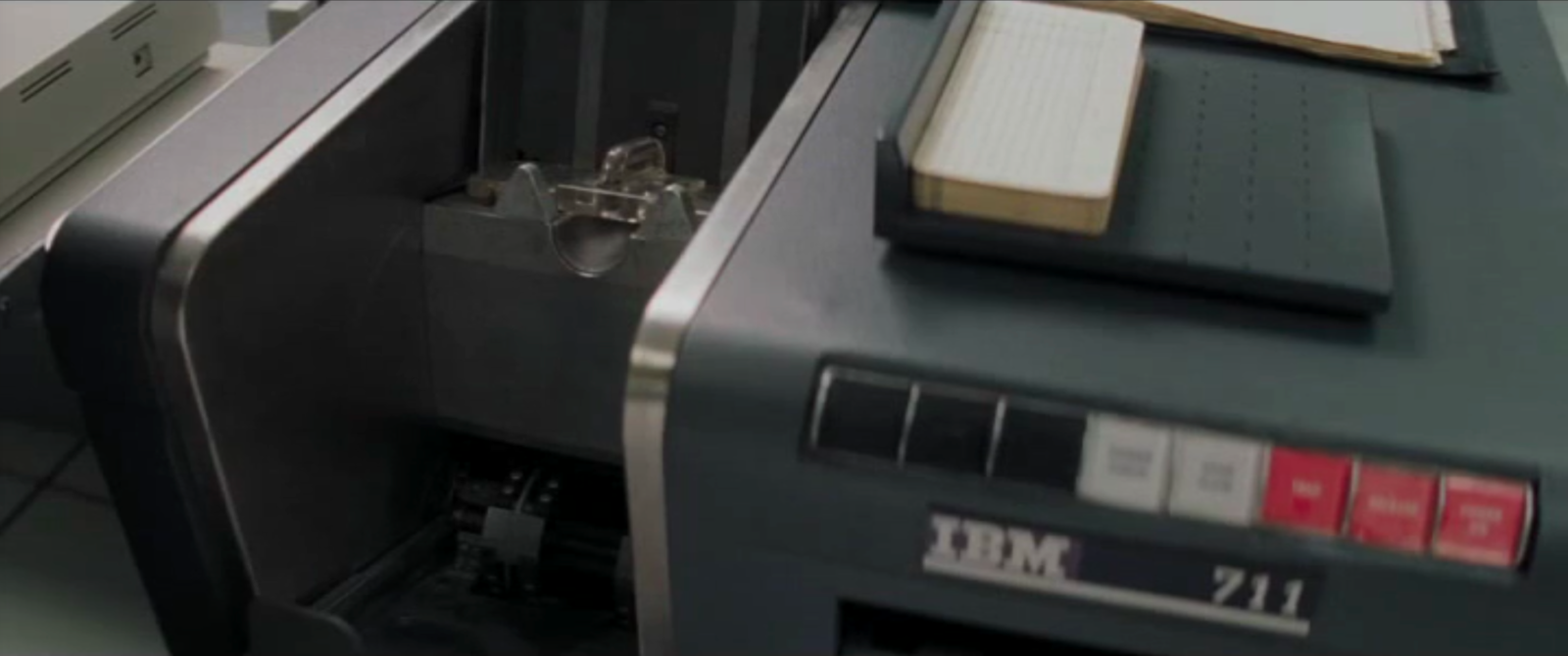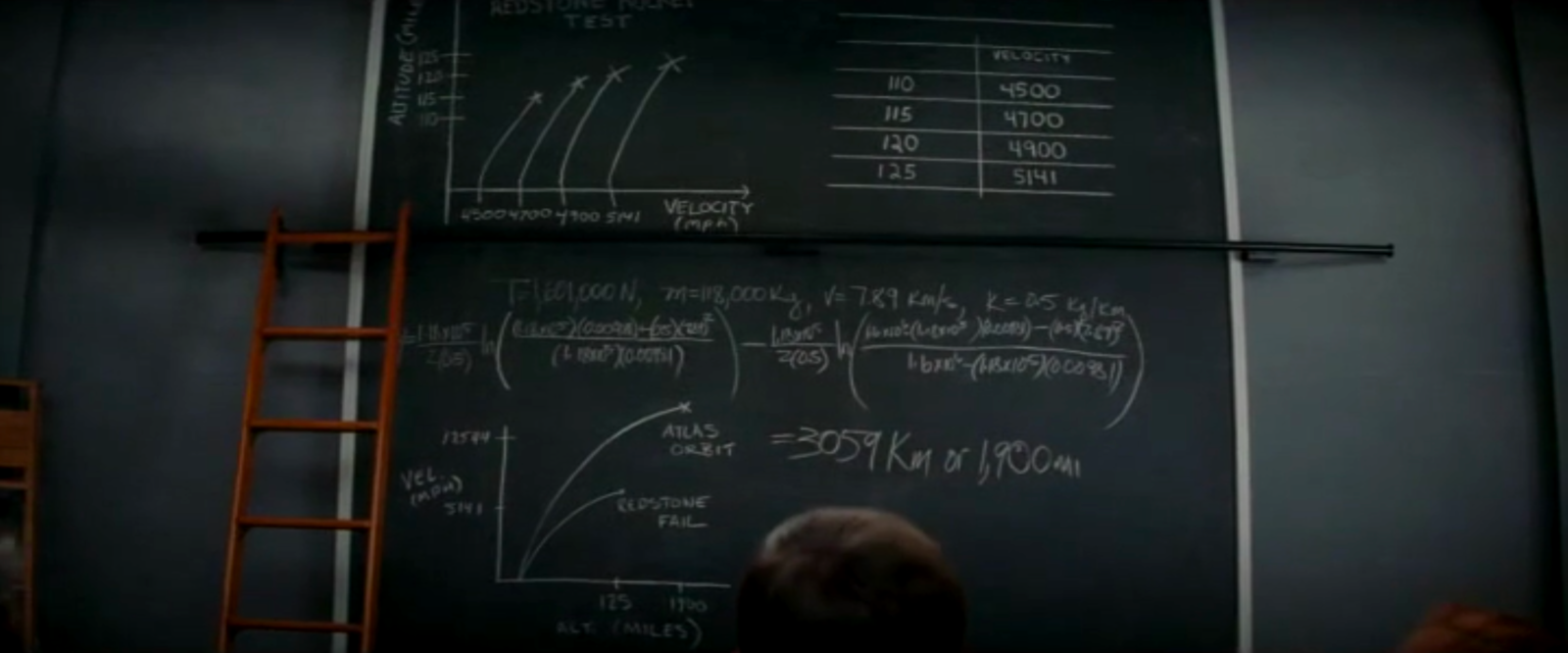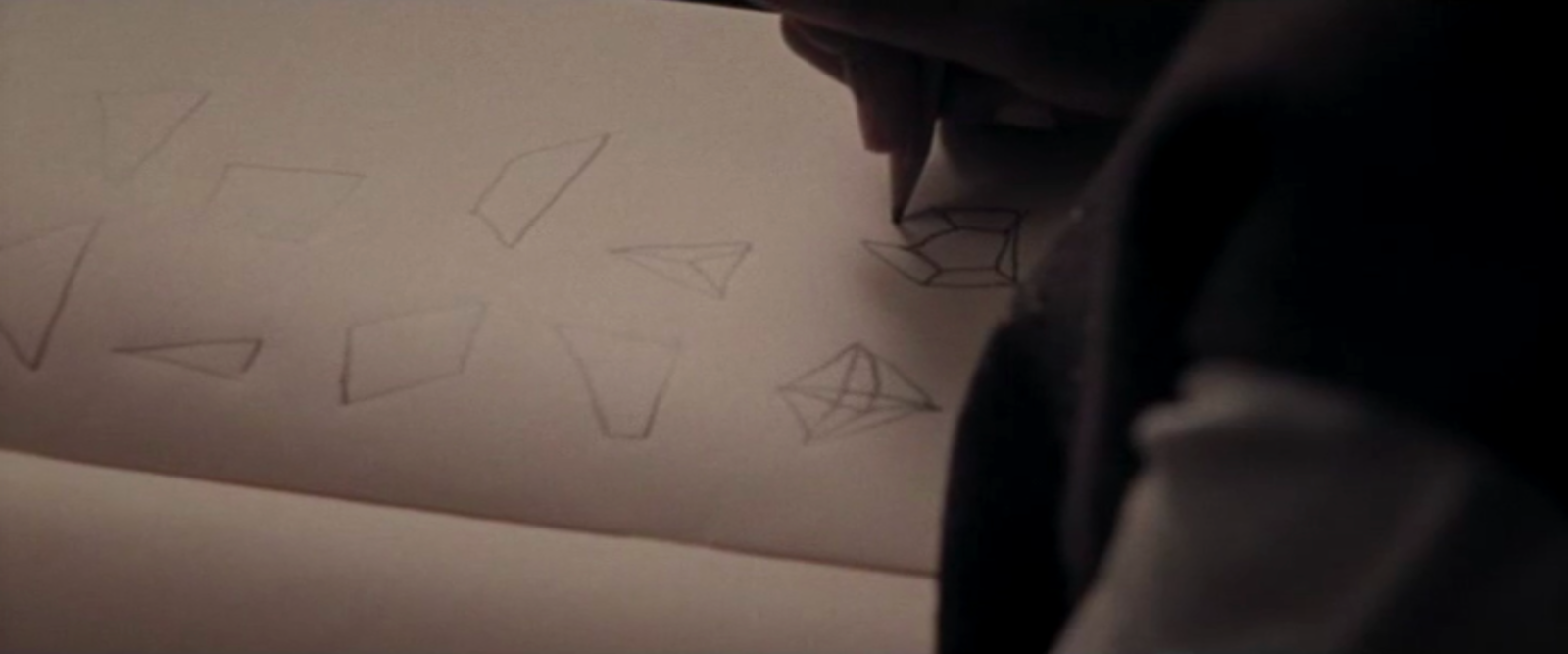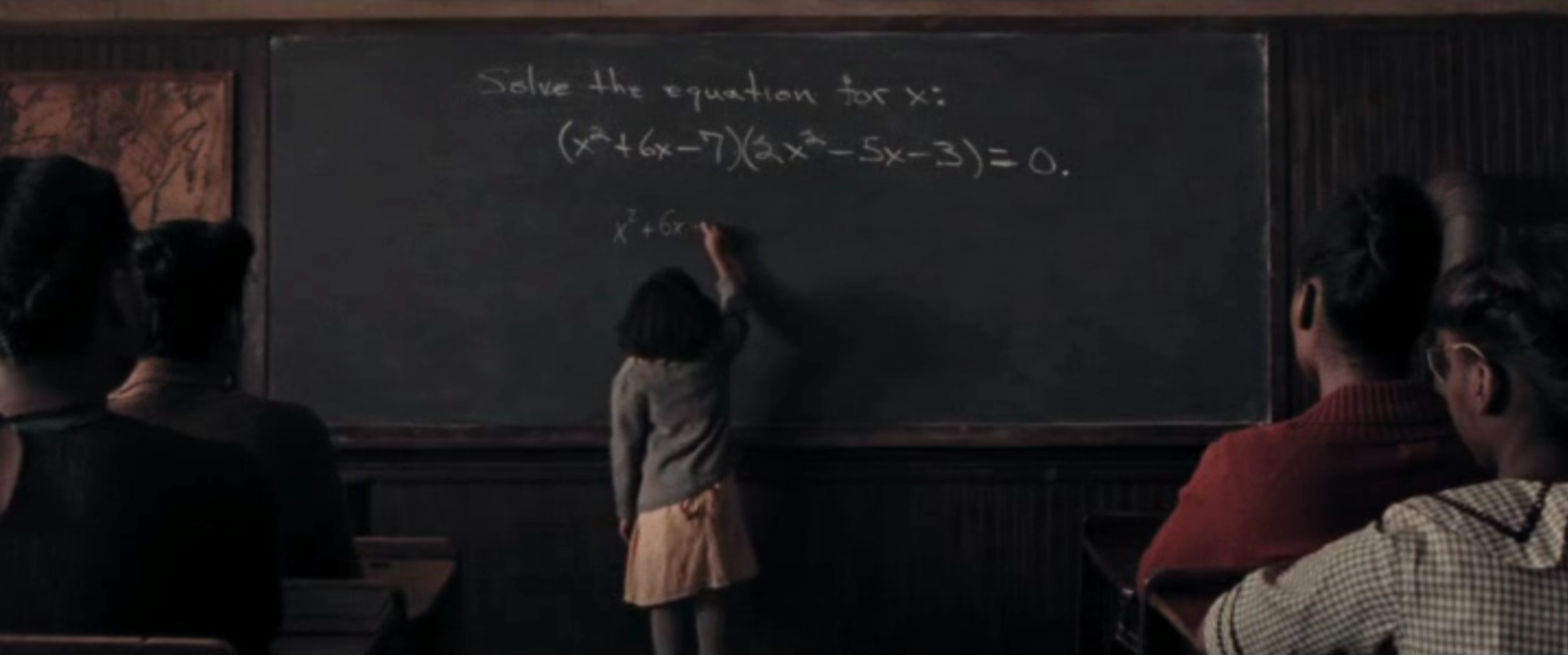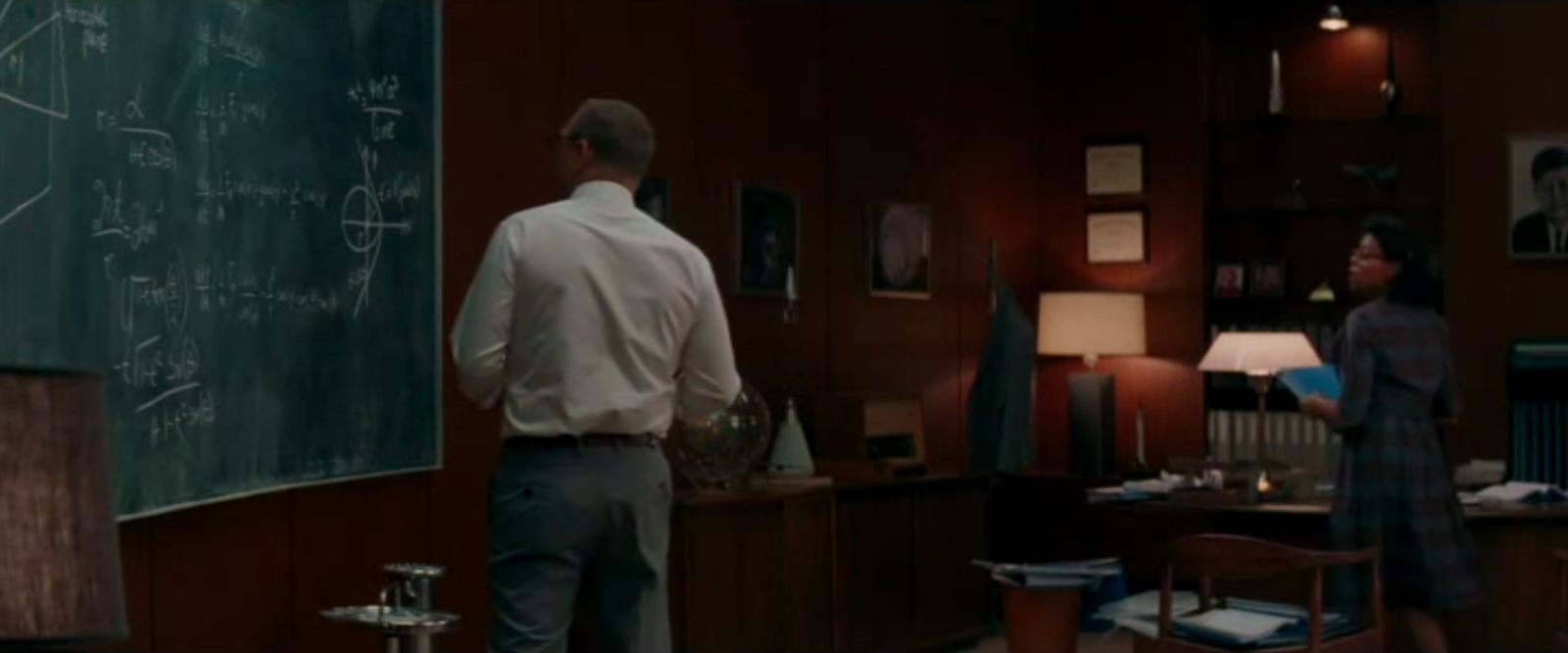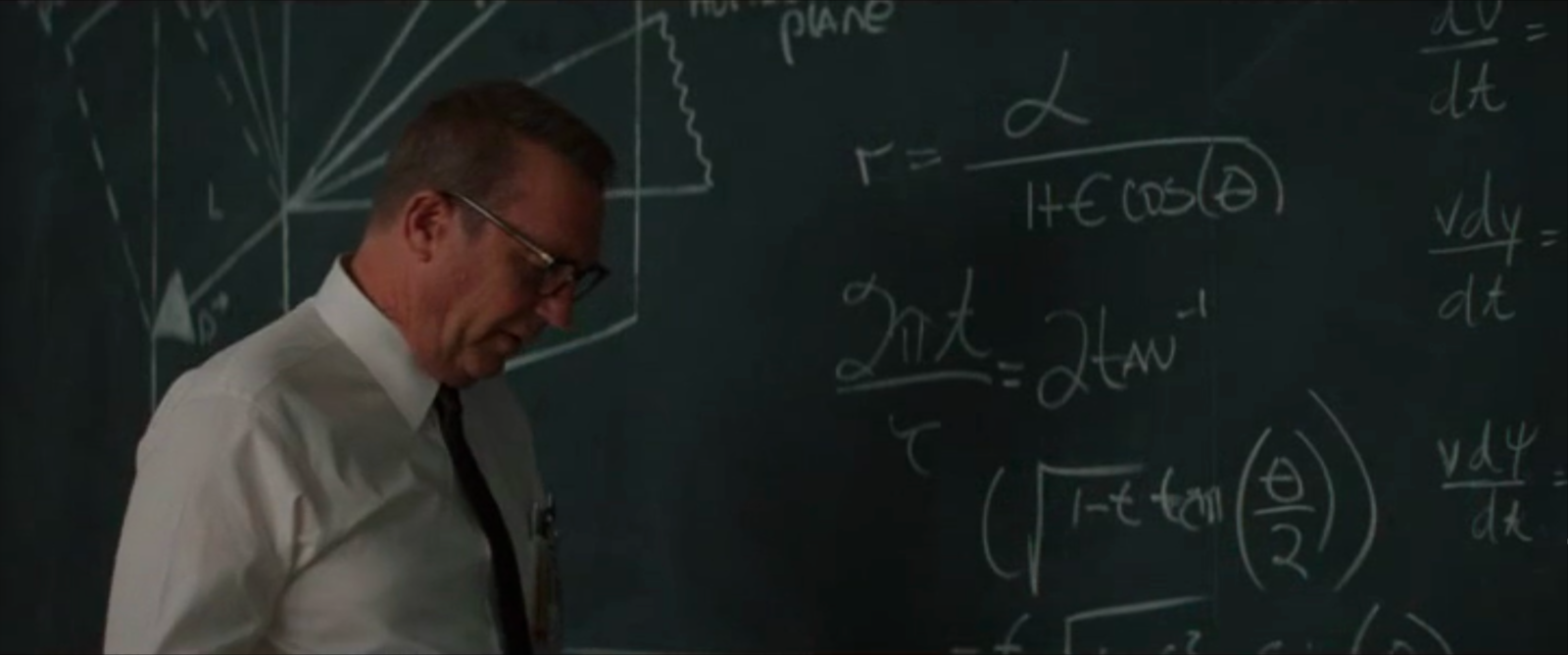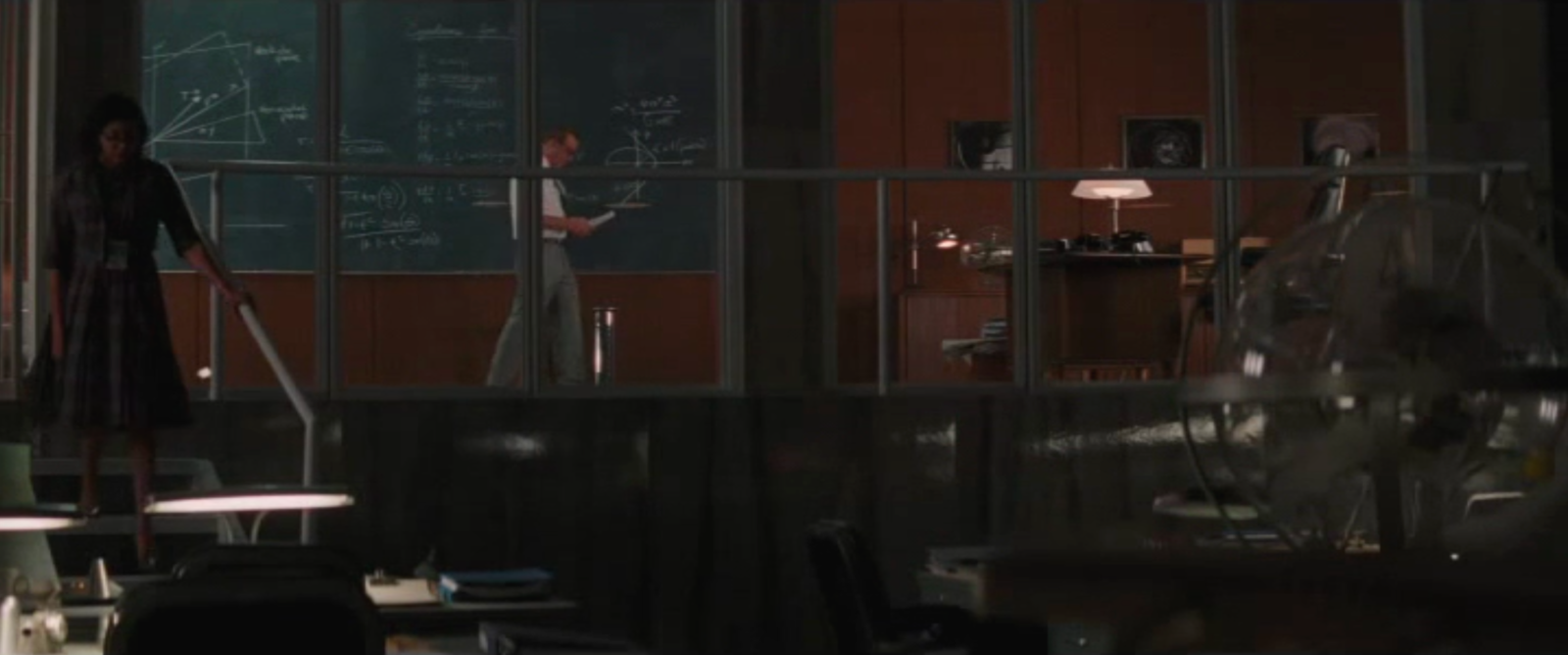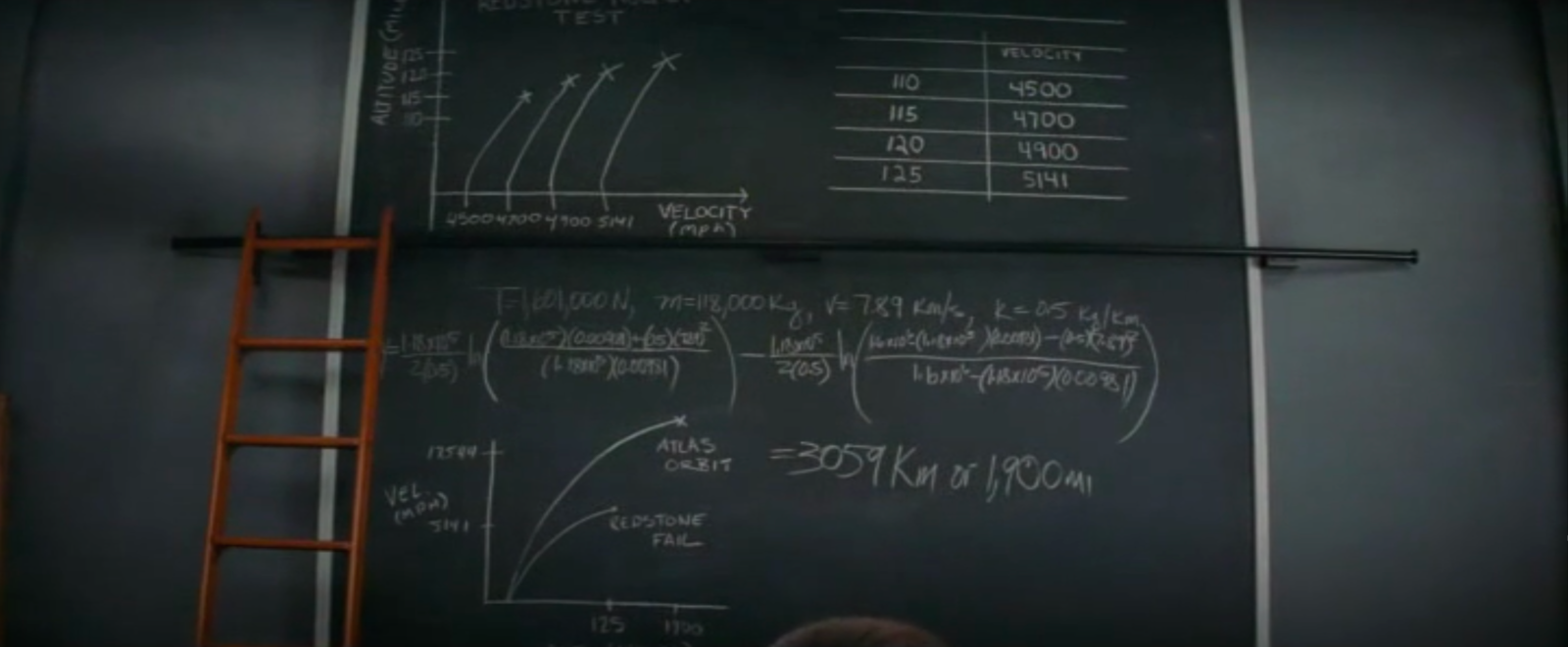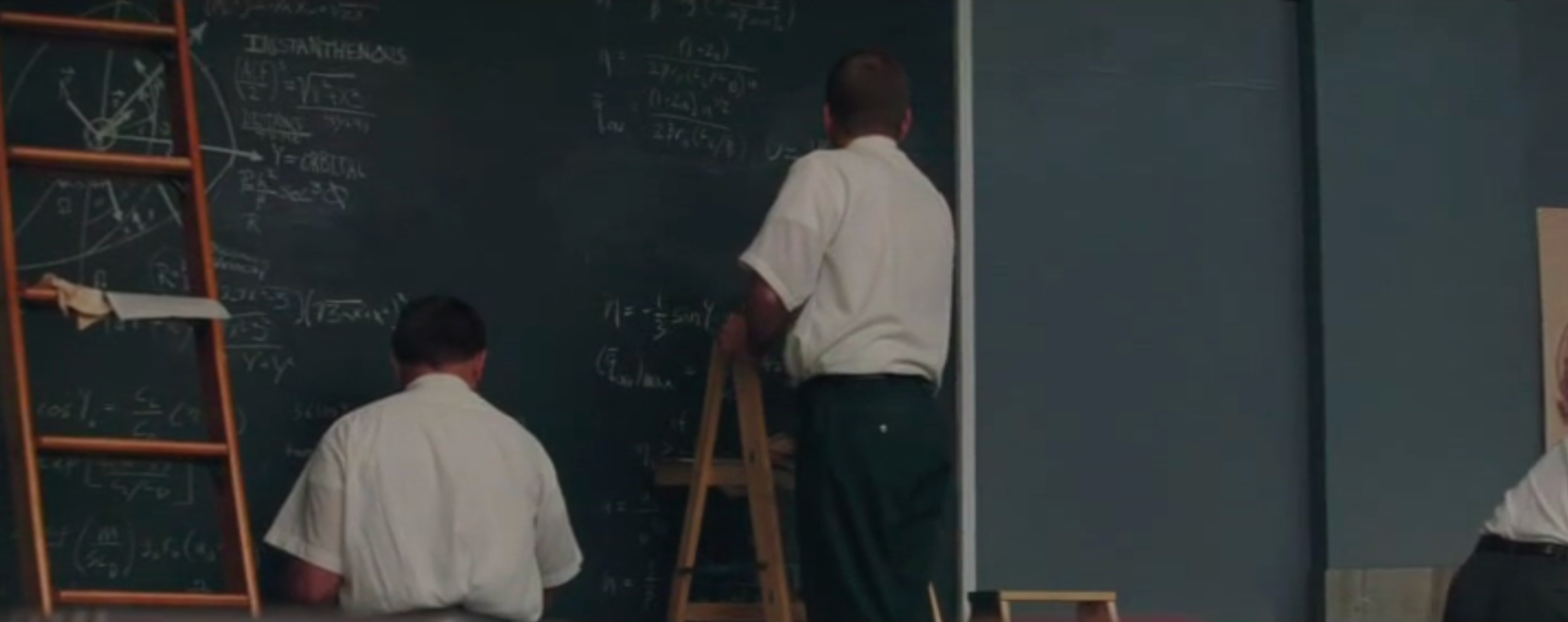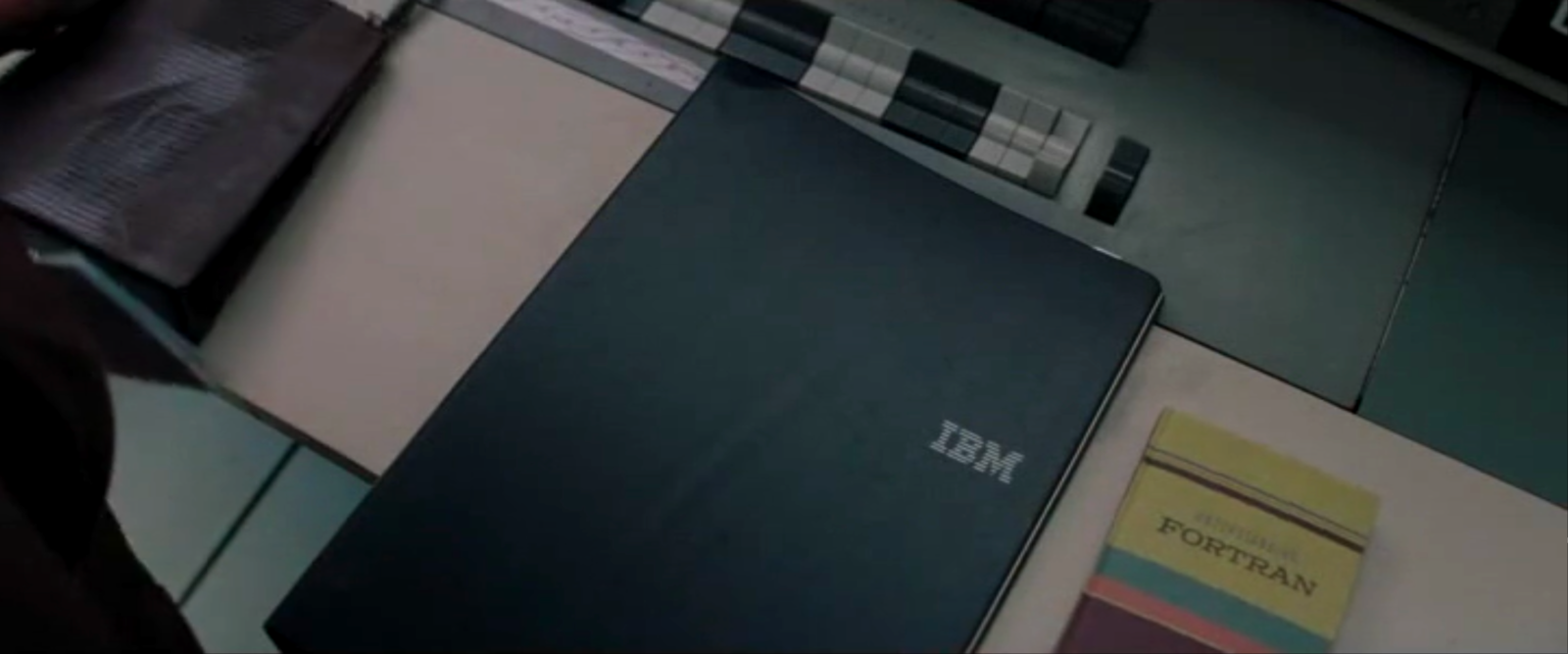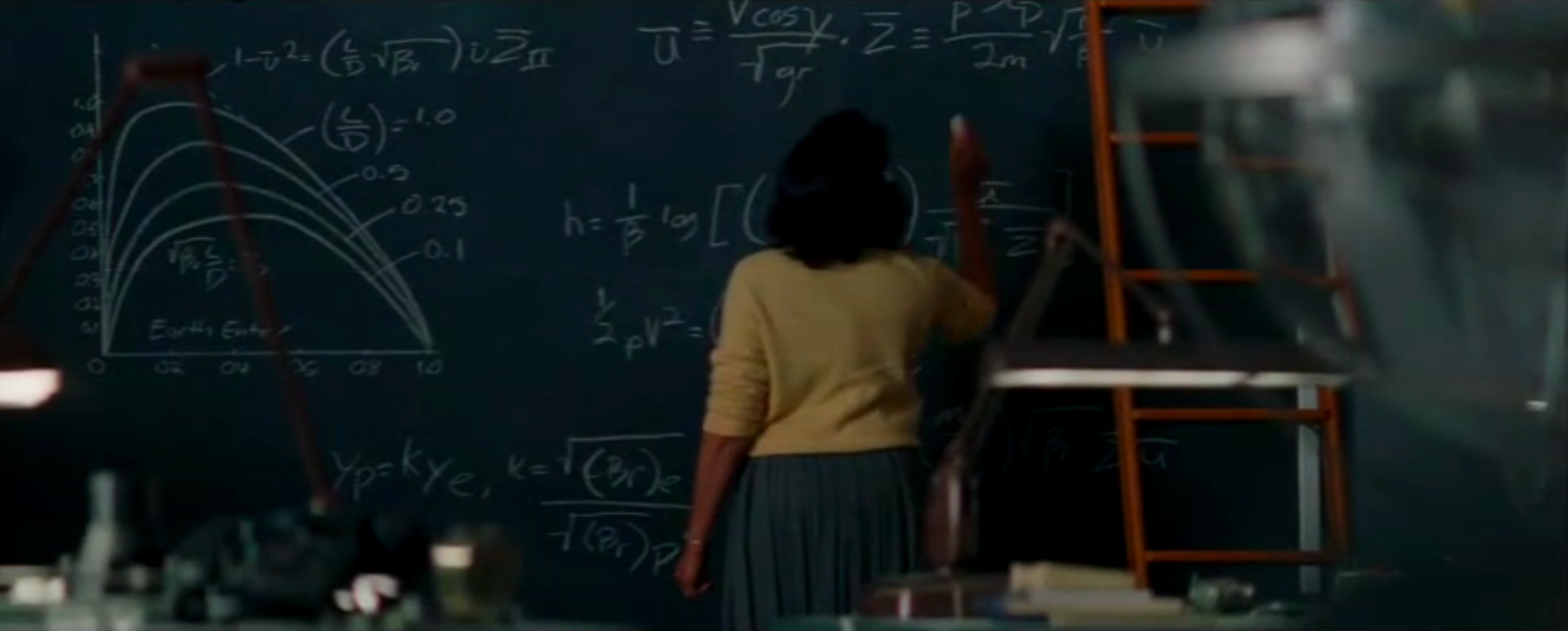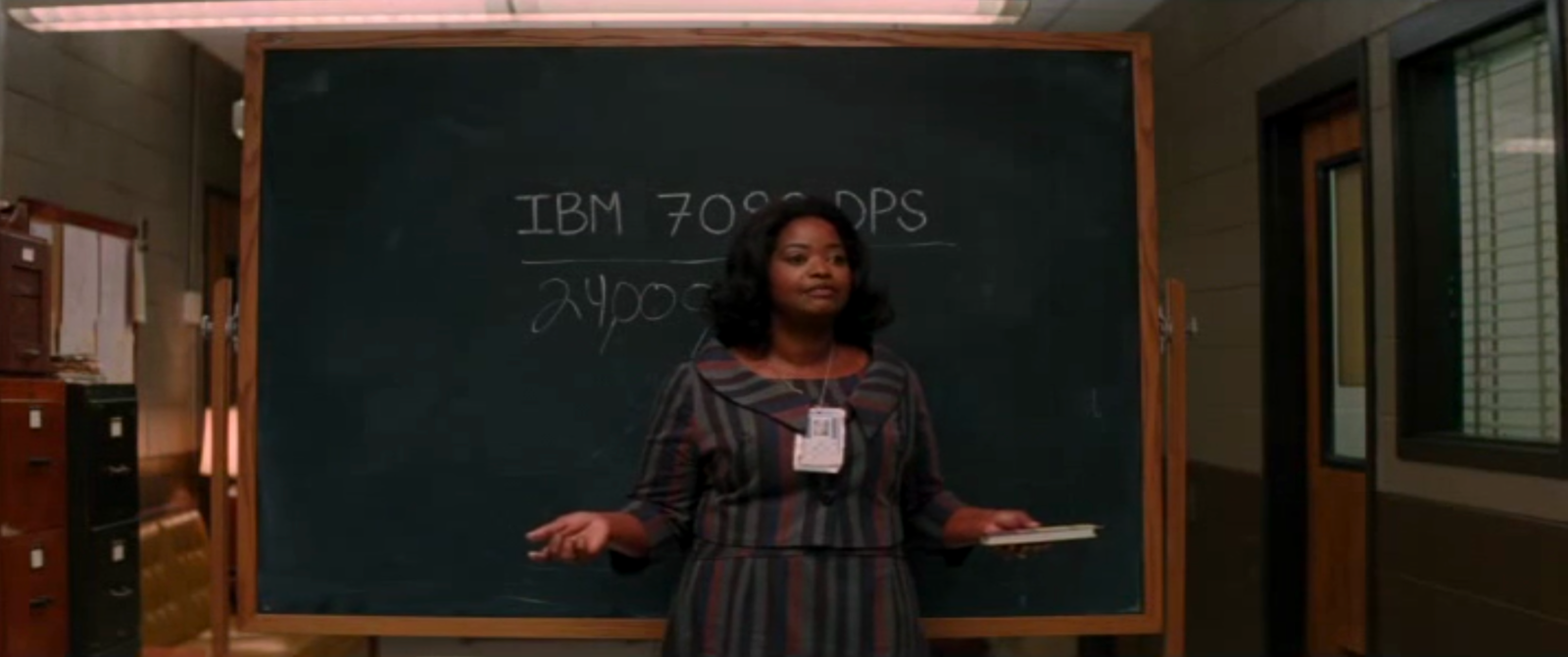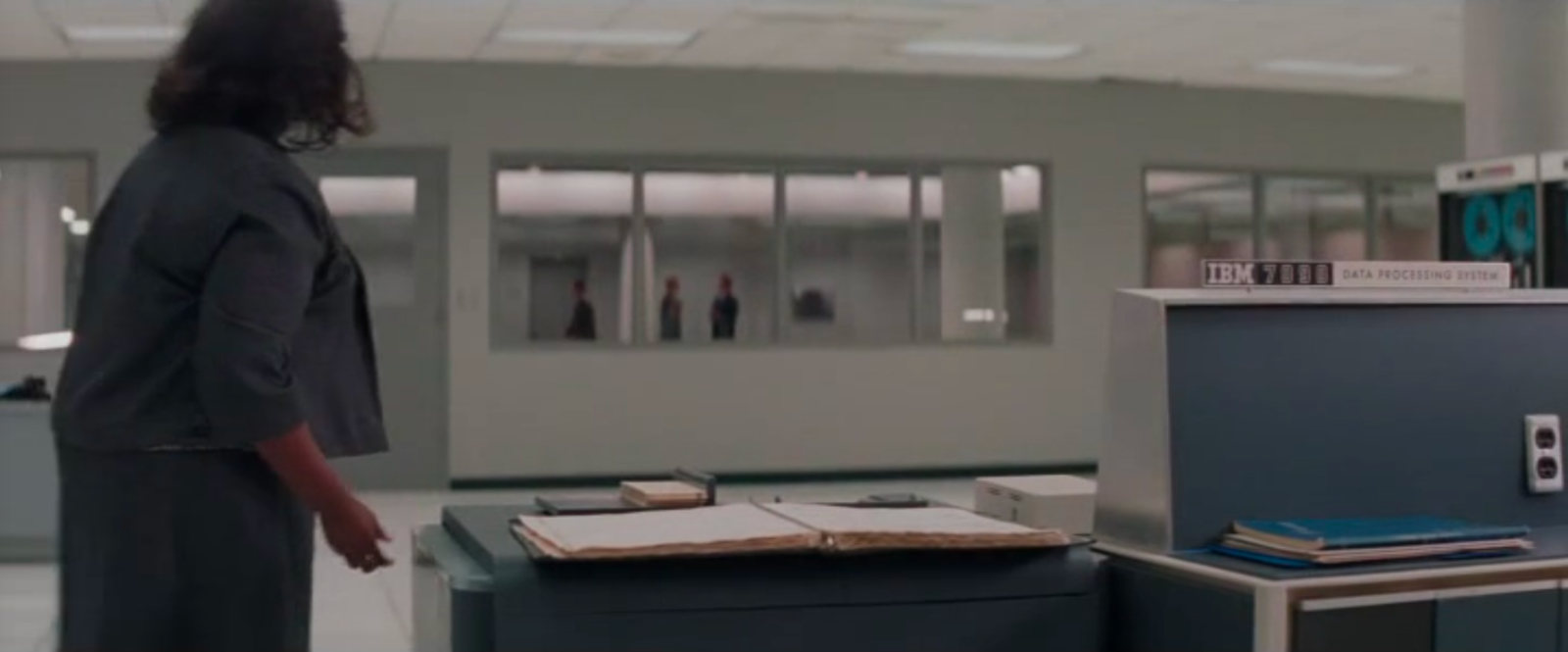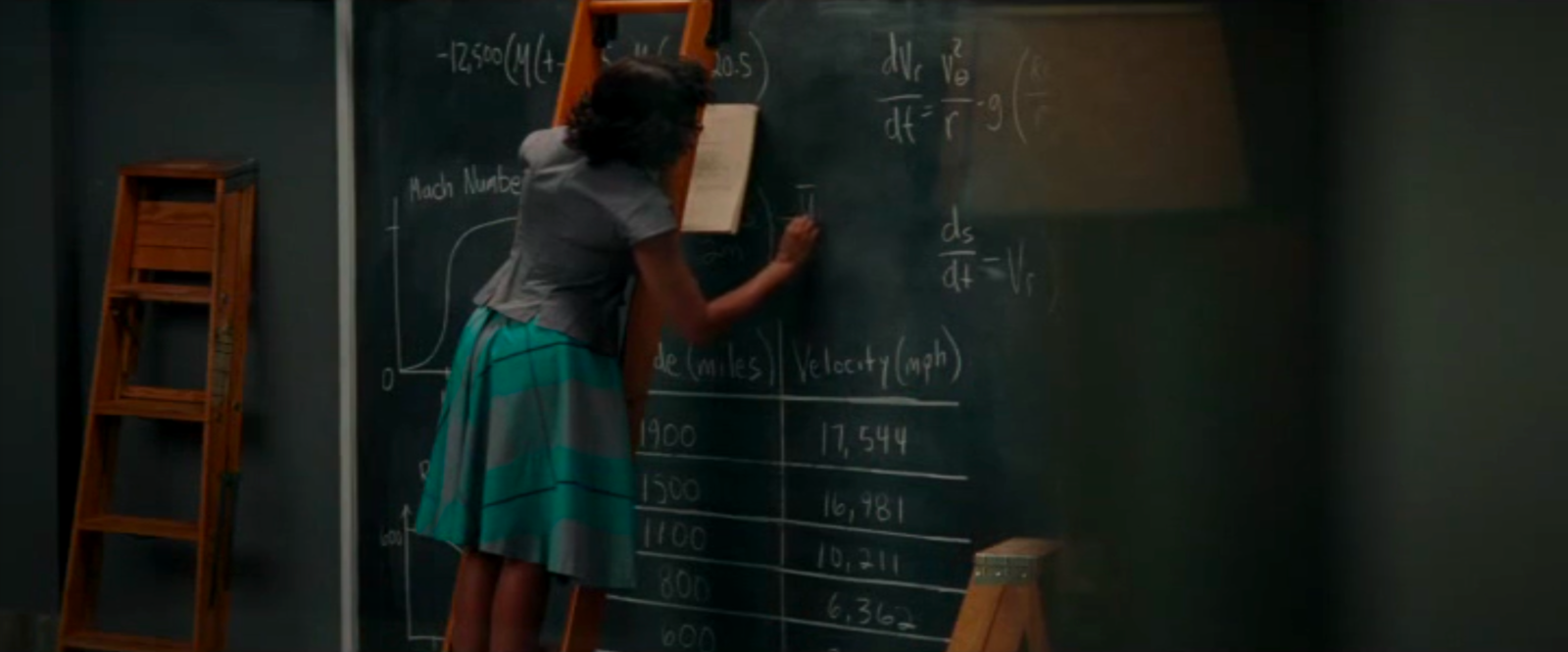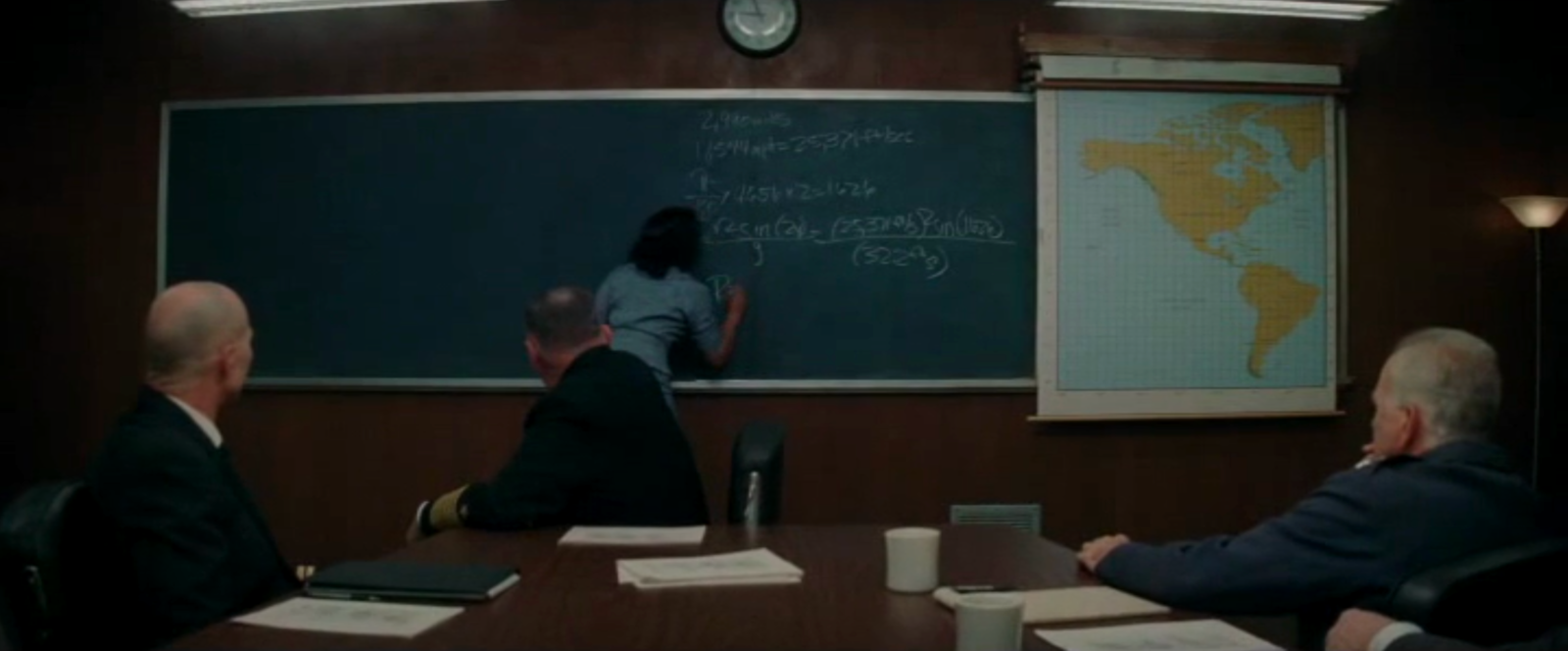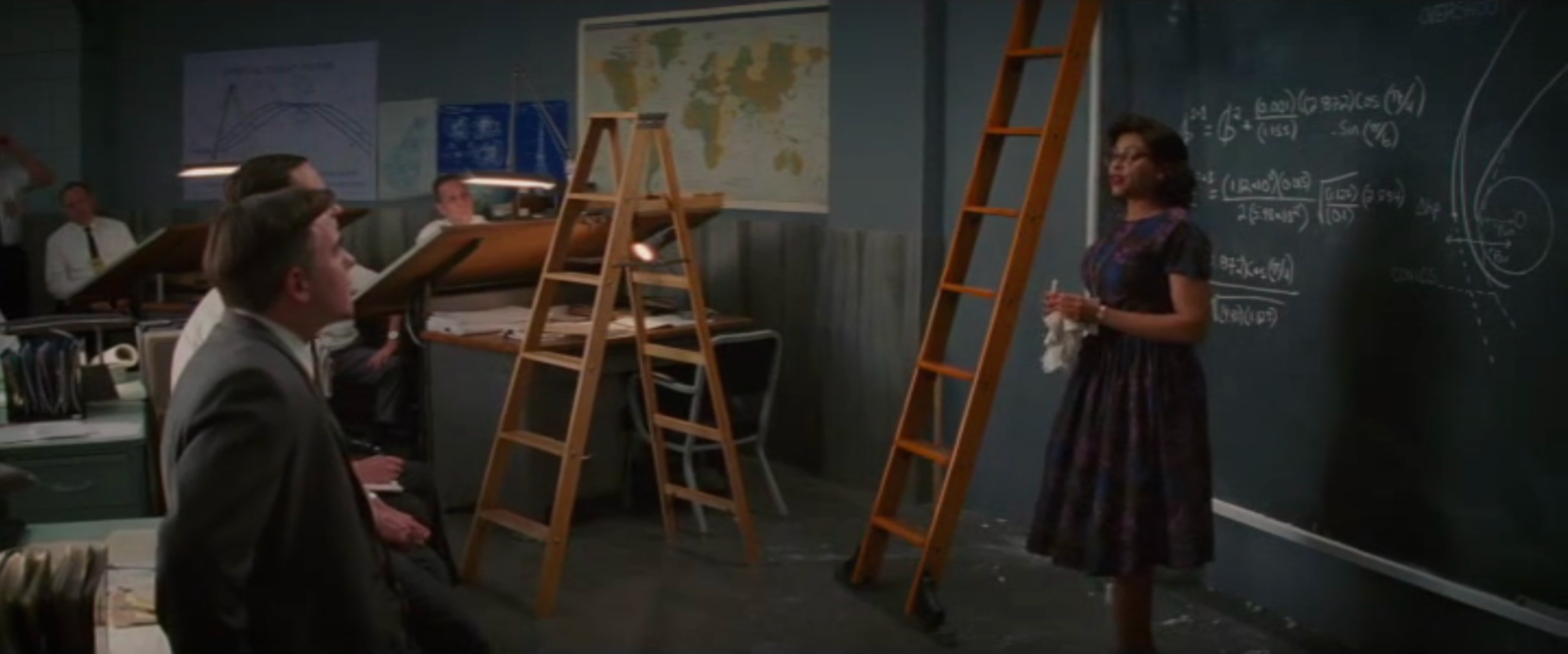M A T H 2 1 B
Mathematics Math21b Spring 2017
Linear Algebra and Differential Equations
Exhibit: Hidden figures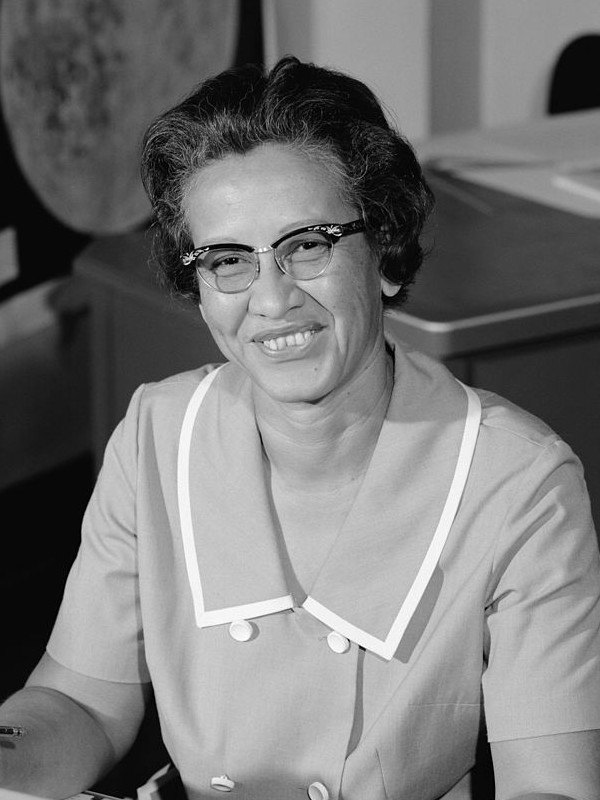Katherine Johnson at NASA 1966. Image Source The new movie Hidden figures features some mathematics. One of the entry scenes in elementary school features a quartic equation ``` (x2 + 6x - 7) (2x2 - 5 x - 3) = 0 ``` Katherine Coleman Goble Johnson (1918 - ) (played by Lidya Jewett as a kid) factors the polynomial. What does this have to do with linear algebra? First of all we will see that characteristic polynomials of matrices are polynomials. We need to factor them in order to find the eigenvalues. We will also see that if we take a polynomial of differential operators, the factorization allows us to solve differential equations. The blackboard of Al Harrison (played by Kevin Costner) features equations of conic sections like ellipses given in polar coordinates as ```r = α/(1+ε cos(θ)) ``` The problem of finding the trajectories of a rocket is a problem in differential equations. One equation we can see ```ds/dt = vr, dvr/dt = vθ2/r g(R/r) ``` An other describes the motion in spherical coordinates (unfortunately, not all formulas are well visible): ```dr/dt = v sin(y) dθ/dt = v cos(y) cos(ψ)/(r cos(φ)) dφ/dt = v cos(y) sin(ψ)/r dv/dt = (1/m) Fr - g sin(y) v dy/dt = (1/m) Fm cos(y) -g cos(y) + (v2/r) cos(y) v dψ/dt = (1/m) F... sin(y) + (v2/2) ``` They are nonlinear but we will cover some nonlinear equations also in our course. Still, the Kepler problem can actually (with some clever transformation) be reduced to a Harmonic oscillator, which is a linear ODE. We see Catherine also work on some differential equations on the large blackboard.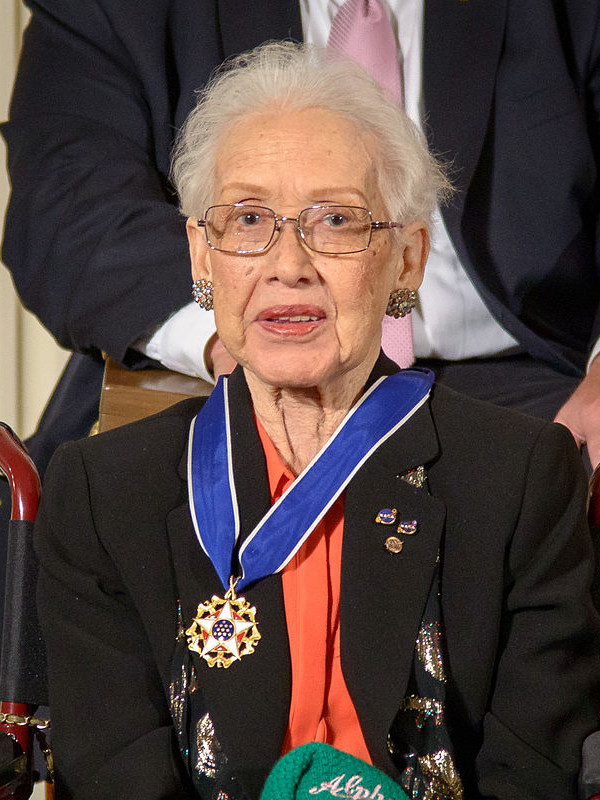Katherine Johnson, 2015 Source